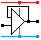Home

rOm
Quest
Glossary

Random
Page
Search
Site
Lush
Sim
Class
Subject
Images

Help
FAQ
Sign
Up
Log
In
 AS Level     Op Amp     Delta Lux     Gain     Schmitt Astable     >Schmitt Trigger<     Virtual Earth     Voltage Follower

# Op Amp Schmitt Trigger

Site for Eduqas/WJEC - Go to the AQA site.

AS Level    Op Amps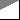Questions 0 to 5   -->  View All

## A Comparator with Different On and Off Voltages

### Uses Positive Feedback

To fully undertand Schmitt triggers you first need to understand ...

• Voltage dividers and the related calculations
• Resistors in parallel and the related calculations
• Op-amp comparator behaviour.

Here is a problem that the Schmitt trigger solves. You have built a circuit to turn on some lights when it gets dark. But when the lights turn on, it gets brighter so the lights go off again. But then it is too dark so they come on again - flashing on and off rapidly.

The Schmitt trigger solves this problem by having different threshold voltages for turning on and off.

When it gets dark, the lights come on. This makes it lighter but not light enough to turn the lights off again. Later when it gets light again, the lights go off. This makes it darker but not dark enough to turn the lights on again.

This behaviour is called hysteresis. The Schmitt trigger uses positive feedback to achieve this effect.

### Simple Inverting Circuit

The input signal is connected to the inverting input.
This is the simplest Schmitt trigger with positive feedback.
It has the advantage of near infinite input impedance.
The switching levels are symetrical because R1 is connected to ground.
For non symetrucal levels, connect R1 to a fixed reference voltage other that zero.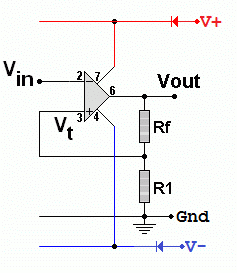• Rf and R1 form a voltage divider.
• Assume that Rf = R1 to make this explanation easier to follow.
• If the Op Amp output is high (say 12 Volts).
The non inverting input will have 6 volts on it.
• The inverting input must rise above 6 Volts to make the op amp output go low.
• The Op Amp output is now low (say -12 Volts).
The non inverting input will have -6 volts on it.
• The inverting input must fall below -6 Volts to make the op amp output go high again.
• By altering Rf and R1, the threshold voltages can be adjusted to solve the problem at hand.

### Alternate Simple Non Inverting Circuit• The input is connected indirectly to the non-inverting input.
• If Vref is zero, the swirching levels will be symetrical about zero volts.
• A simple design trick to calculate the resistors is to have 10k represent 1 volt. Then there is minimal maths to do.
• If the op-amp saturates at +/- 10 volts ( typical for 12 volt power lines ) make Rf equal to 100k.
• If you want 5 volt switching levels, make R1 equal to 50k,
• You could select 5 and 10k but the input current would be unnecessarily greater.
• You could select 500k and 1MΩ but op-amp leakage currents might be enough to disturb the switching levels.

### A More Versatile Inverting Circuit

This is a comparator converted into a Schmitt trigger by adding one positive feedback resistor to give different on and off switching levels.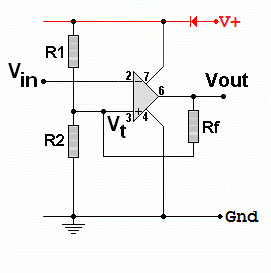• If the output is low, Rf is in parallel with R2.
The combined resistance can be calculated using the resistors in parallel formula.
• If the output is high, Rf is in parallel with R1.
The combined resistance can be calculated using the resistors in parallel formula.
• The lower threshold voltage is found using the voltage divider formula with R2 in parallel with Rf.
• The upper threshold voltage is found using the voltage divider formula with R1 in parallel with Rf.
• As it's an inverting Schmitt trigger, a low input gives a high output and vice versa.
• If R1 and R2 are equal, the switching levels will be symetrical. Non equal values give you more control over the switching levels.

### Oscilloscope plot showing the Voltage Transfer Characteristic of the Schmitt Trigger

• When the blue trace drops below -6V the red trace goes high.
• When the blue trace rises above 6V the red trace goes low.### Noise Clean Up

As this is an inverting Schmitt Trigger, the output pulse is inverted relative to the input. Here you can see a very noisy input that would produce errors in four or five places with a simple comparator using the grey line reference voltage. The Schmitt trigger has two reference voltages. The input must go above the red line for a low output and below the blue line for a high output. This removes all noise unless the noise signal is so large that it exceeds the difference between the reference voltages. Although the amplitude noise is removed, there is still some timing noise called Jitter. The pulses might switch slightly too soon or too late. This can be removed with other digital techniques beyond A Level scope.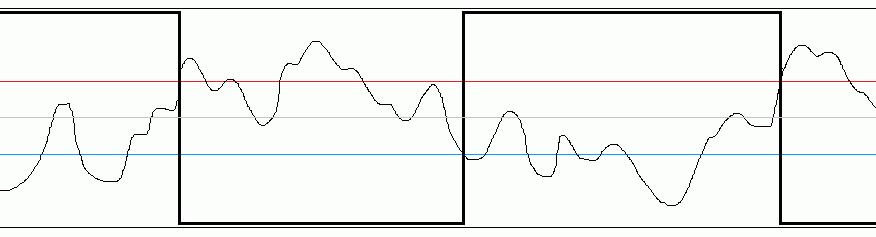### Single power rail simple Inverting Schmitt Trigger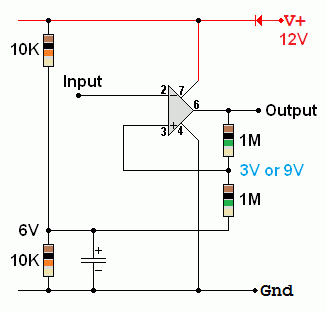This uses a voltage divider to produce a six volt level.
The upper and lower switching levels in this exmple are 3V and 9V.
The feedback resistors need to be much larger than the voltage divider resistors for this circuit to behave well.

Subject Name     Level     Topic Name     Question Heading     First Name Last Name Class ID     User ID

 CloseEscapeX Q: qNum of last_q     Q ID: Question ID         Score: num correct/num attempts         Date Done

Question Text

image url

Help Text
Debug

• You can attempt a question as many times as you like.
• If you are logged in, your first attempt, each day, is logged.
• To improve your scores, come back on future days, log in and re-do the questions that caused you problems.
• In the grade book, you can delete your answers for a topic before re-doing the questions. Avoid deleting unless you intend re-doing the questions very soon.